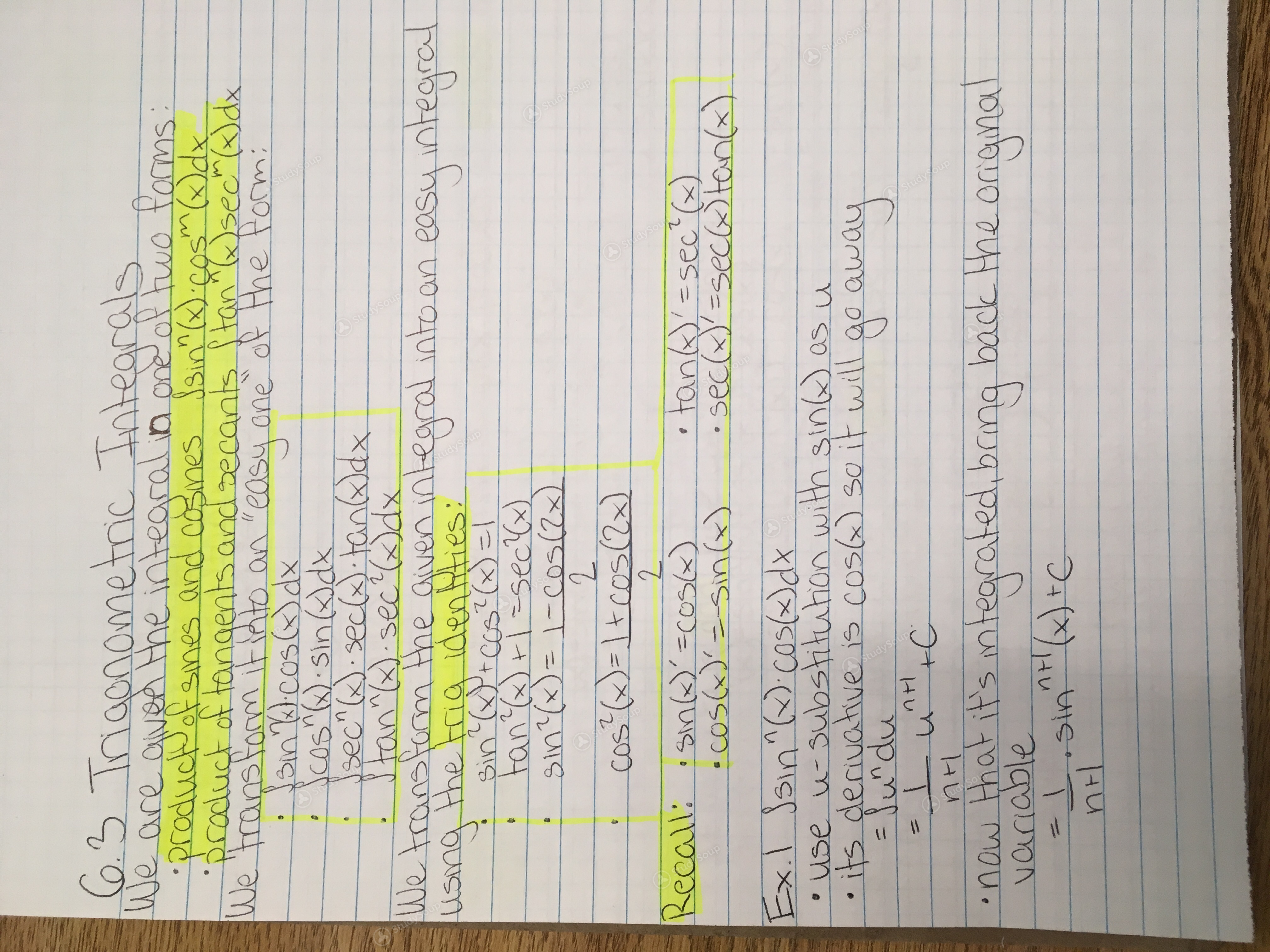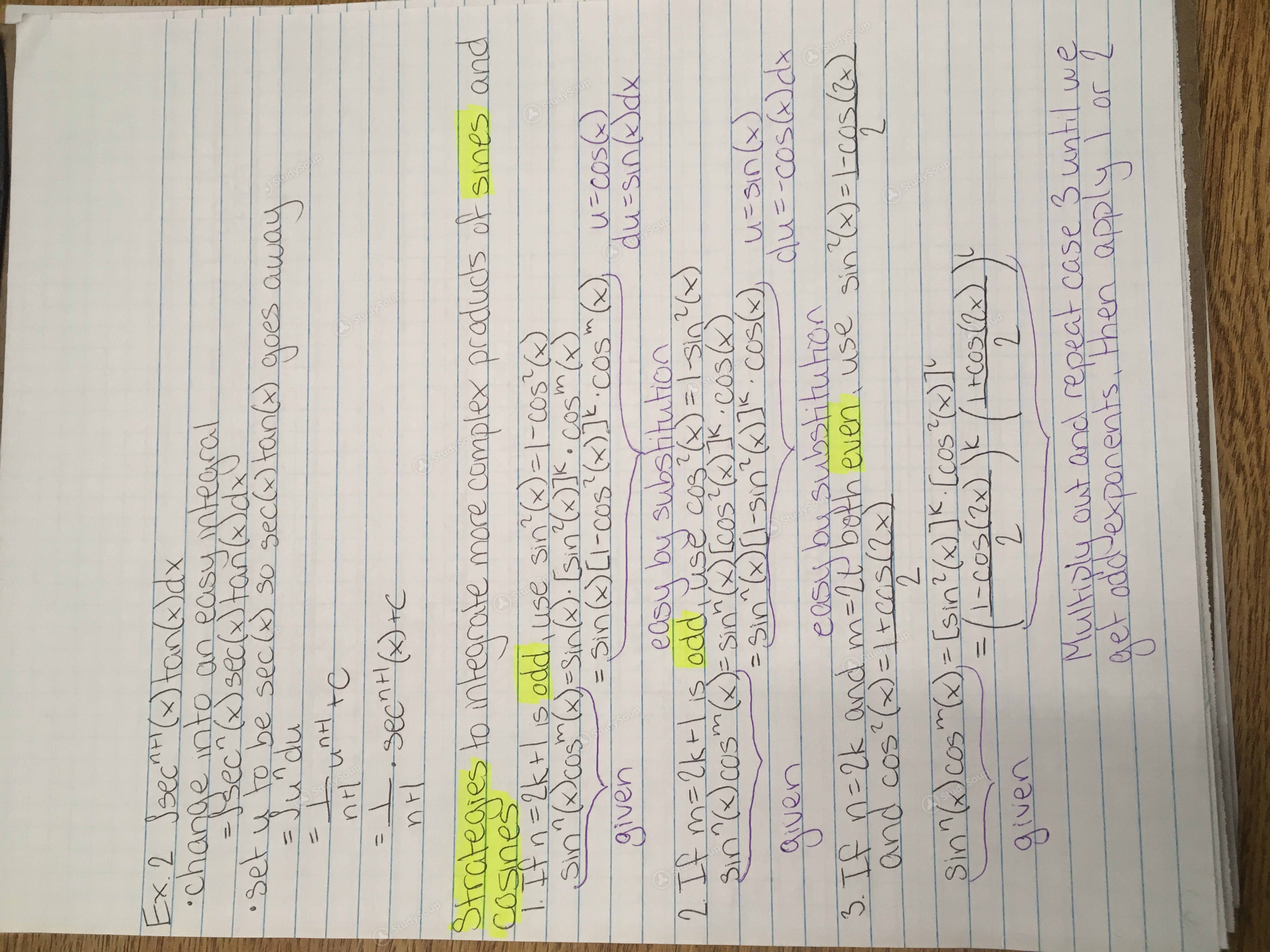Limited time offer 20% OFF StudySoup Subscription details

# UCR - MATH 009B - Math 9B, Week 5 - Class Notes

### Created by: Amber Elite Notetaker

> > > > UCR - MATH 009B - Math 9B, Week 5 - Class Notes

UCR - MATH 009B - Math 9B, Week 5 - Class Notes

##### Uploaded: 11/18/2018
This preview shows pages 1 - 2 of a 2 page document. to view the rest of the contentThis is the end of the preview. Please to view the rest of the contentJoin more than 18,000+ college students at University of California Riverside who use StudySoup to get ahead
2 Pages 29 Views 23 Unlocks
• Better Grades Guarantee
• 24/7 Homework help
• Notes, Study Guides, Flashcards + More!Join more than 18,000+ college students at University of California Riverside who use StudySoup to get ahead
##### Uploaded: 11/18/2018
2 Pages 29 Views 23 Unlocks
• Better Grades Guarantee
• 24/7 Homework help
• Notes, Study Guides, Flashcards + More!

Unformatted text preview: 6.3 Trigonometric Integrals We are given the integral in one of two forms _product of sines and cosines sin" (x) cosm @dx Product of tangents and secants stan sech ()dx We transform it into an easy one of the form Binu.cos(x) dx Scos"Q) sin @dx Stan sea tan@dx 2 We transform the given integral into an easy integral using the Fria identties: ? Sin? QP+cos? (x) = tan?(x) =sec (x) sin?(x)= 1-cos(2x) cos?Q=1tcos(2x) 2 Recalli: :sing)'cosc) 'tan 'esec() cos(x)=sin(x) sec(x)=secatan(x) Ex.l jsin^(.cos(x)dx use u-substitution with sin@) as u .its derivative is cos@ so it will go away = fundu - Lutte now that it's integrated, bang back the original variable = sinnt (x) +CEx. 2 Ssecni tandx chanele into an easy integral slundu set y to be seca so seca tan@ goes away it und te int- seen HD+C Strategies to integrate more complex products of sines and cosines 1. If n = 2k+ is odd, use sin" ()= |-C05 sin" (cos(x)=sin). (sin Wyk. Cosmo = sin() [l-cos (x)]k.cos" @ u-cos given duEsindx easy by substitution 2. If m=2K+i oddy use cos?) = | sin ) 3in" (cos(x)=sin([cos(x)]k.cos) a sin^@[l-sin? (6)]5. cos() uasin (. given du e-cos@dx easy bu substitution 3. If n = 2k and m=2P both even. use sin Q)-l-cos(2) and cos? () = 1cos(2x) Sin"@cosm()= [sin?(x)]K. [cos?_)]' = l 1-cos(2x) * ( cos(2x)) 2 given weEx3 Scos (2x) dx 3 is odd 30 we separate one cosine and transform all other cosines to sines using cos? (0) - I-sin? (0) = s cos(2x) cos?(2x) dx = 3 cos (2x) [i-sin?(2x)]dx apply u-substitution u=sin (2x) du-cos(lx) 2dx 42 du=cos (2x) dx = ] Il-? 1.72 du integrate as usual now that it's usingu - Uz S(1-3 du osplit yms. (I-updu into two integrals =Y2E5ldu- su du] 542 Sidu-Y2 Surdu =Y 2.-42:13u> rc replace u with the original variable =Y2 Sin (2x) - Yousins () +C Ex.4 Isip .cos@dx :3 is odd so we separate one sine, and turn all other = sin(x) sin?X.cos(xclx = Isina). [l-cos?)].cos? @dx. apply u-substitution du=-sin(x) dx -du= sindx. u=00SX ay u Brys us to --/3 cos@+y5.cos W C

Join StudySoup for FREE
Get Full Access to UCR - Class Notes - Week 5
Already have an account? Login here
×
Log in to StudySoup
Get Full Access to UCR - Class Notes - Week 5

Forgot password? Reset password here

Reset your password

I don't want to reset my password

Need help? Contact support

Need an Account? Is not associated with an account
We're here to help

Having trouble accessing your account? Let us help you, contact support at +1(510) 944-1054 or support@studysoup.com

Password Reset Request Sent An email has been sent to the email address associated to your account. Follow the link in the email to reset your password. If you're having trouble finding our email please check your spam folder
Already have an Account? Is already in use
Incorrect Password The password used to log in with this account is incorrect

Forgot password? Reset it here